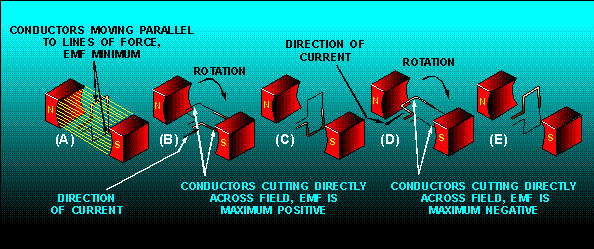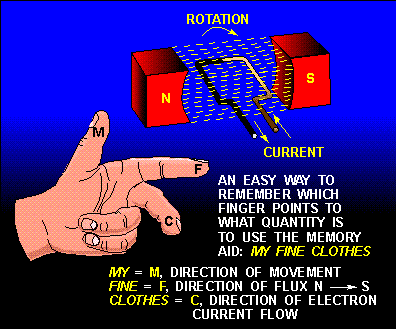magnetic field produces an emf in a conductor. That is, if a conductor is placed in a magnetic field, and either the field or the conductor moves, an emf is induced in the conductor.">Custom SearchBASIC AC GENERATION From the previous discussion you learned that a current-carrying conductor produces a magnetic field around itself. In module 1, under producing a voltage (emf) using magnetism, you learned how a changing magnetic field produces an emf in a conductor. That is, if a conductor is placed in a magnetic field, and either the field or the conductor moves, an emf is induced in the conductor. This effect is called electromagnetic induction. CYCLE Figures 1-7 and 1-8 show a suspended loop of wire (conductor) being rotated (moved) in a clockwise direction through the magnetic field between the poles of a permanent magnet. For ease of explanation, the loop has been divided into a dark half and light half. Notice in (A) of the figure that the dark half is moving along (parallel to) the lines of force. Consequently, it is cutting NO lines of force. The same is true of the light half, which is moving in the opposite direction. Since the conductors are cutting no lines of force, no emf is induced. As the loop rotates toward the position shown in (B), it cuts more and more lines of force per second (inducing an ever-increasing voltage) because it is cutting more directly across the field (lines of force). At (B), the conductor is shown completing one-quarter of a complete revolution, or 90, of a complete circle. Because the conductor is now cutting directly across the field, the voltage induced in the conductor is maximum. When the value of induced voltage at various points during the rotation from (A) to (B) is plotted on a graph (and the points connected), a curve appears as shown below. Figure 1-7. - Simple alternating-current generator.Figure 1-8. - Basic alternating-current generator.As the loop continues to be rotated toward the position shown below in (C), it cuts fewer and fewer lines of force. The induced voltage decreases from its peak value. Eventually, the loop is once again moving in a plane parallel to the magnetic field, and no emf is induced in the conductor. The loop has now been rotated through half a circle (one alternation or 180). If the preceding quarter-cycle is plotted, it appears as shown below. When the same procedure is applied to the second half of rotation (180 through 360), the curve appears as shown below. Notice the only difference is in the polarity of the induced voltage. Where previously the polarity was positive, it is now negative. The sine curve shows the value of induced voltage at each instant of time during rotation of the loop. Notice that this curve contains 360, or two alternations. TWO ALTERNATIONS represent ONE complete CYCLE of rotation. Assuming a closed path is provided across the ends of the conductor loop, you can determine the direction of current in the loop by using the LEFT-HAND RULE FOR GENERATORS. Refer to figure 1-9. The left-hand rule is applied as follows: First, place your left hand on the illustration with the fingers as shown. Your THUMB will now point in the direction of rotation (relative movement of the wire to the magnetic field); your FOREFINGER will point in the direction of magnetic flux (north to south); and your MIDDLE FINGER (pointing out of the paper) will point in the direction of electron current flow. Figure 1-9. - Left-hand rule for generators.By applying the left-hand rule to the dark half of the loop in (B) in figure 1-8, you will find that the current flows in the direction indicated by the heavy arrow. Similarly, by using the left-hand rule on the light half of the loop, you will find that current therein flows in the opposite direction. The two induced voltages in the loop add together to form one total emf. It is this emf which causes the current in the loop. When the loop rotates to the position shown in (D) of figure 1-8, the action reverses. The dark half is moving up instead of down, and the light half is moving down instead of up. By applying the left-hand rule once again, you will see that the total induced emf and its resulting current have reversed direction. The voltage builds up to maximum in this new direction, as shown by the sine curve in figure 1-8. The loop finally returns to its original position (E), at which point voltage is again zero. The sine curve represents one complete cycle of voltage generated by the rotating loop. All the illustrations used in this chapter show the wire loop moving in a clockwise direction. In actual practice, the loop can be moved clockwise or counterclockwise. Regardless of the direction of movement, the left-hand rule applies. If the loop is rotated through 360 at a steady rate, and if the strength of the magnetic field is uniform, the voltage produced is a sine wave of voltage, as indicated in figure 1-9. Continuous rotation of the loop will produce a series of sine-wave voltage cycles or, in other words, an ac voltage. As mentioned previously, the cycle consists of two complete alternations in a period of time. Recently the HERTZ (Hz) has been designated to indicate one cycle per second. If ONE CYCLE PER SECOND is ONE HERTZ, then 100 cycles per second are equal to 100 hertz, and so on. Throughout the NEETS, the term cycle is used when no specific time element is involved, and the term hertz (Hz) is used when the time element is measured in seconds. Q.16 When a conductor is rotated in a magnetic field, at what points in the cycle is emf (a) at maximum amplitude and (b) at minimum amplitude? Q.17 One cycle is equal to how many degrees of rotation of a conductor in a magnetic field? Q.18 State the left-hand rule used to determine the direction of current in a generator. Q.19 How is an ac voltage produced by an ac generator?Integrated Publishing, Inc. - A (SDVOSB) Service Disabled Veteran Owned Small Business